# Continuous mapping

A mappingfrom a topological spaceinto a topological spacesuch that for every pointand for every neighbourhood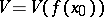of its image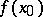there is a neighbourhoodofsuch the. This definition is a rephrasing of the neighbourhood definition of continuity of a function of a real variable (see Continuous function). There are many equivalent definitions of continuity. Thus, for the continuity of a mappingit is necessary and sufficient that any one of the following conditions holds:

a) the inverse imageof every open setinis open in;

b) the inverse image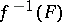of every closed setinis closed in;

c)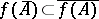for every set(the image of the closure is contained in the closure of the image).

The concept of a continuous function, which was correctly stated already by B. Bolzano and A.L. Cauchy, played an important role in the mathematics of the 19th century. Weierstrass' function, which is nowhere differentiable, "Cantor's staircase" and Peano's curve pointed to the need of considering more special cases of continuity. The necessity of selecting special classes of mappings became even more urgent when continuous mappings of more general objects — topological spaces — were considered. One could mention the following important types of continuous mappings: topological mappings or homeomorphisms, perfect mappings, closed mappings, open mappings, and quotient mappings (cf. Homeomorphism; Closed mapping; Open mapping; Perfect mapping; Quotient mapping). Ifand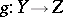are two continuous mappings, then their composite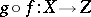that is, the mapping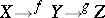is also continuous. Every identity mapping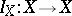is evidently continuous. Therefore, topological spaces and continuous mappings form a category.

One of the aims of topology is the classification of both spaces and mappings. Its essence consists of the following: three fundamental and closely connected problems are selected. 1) In what case can every space of a certain fixed classbe mapped into some space of a class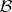by a continuous mapping belonging to a class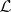? 2) By what intrinsic properties can one characterize spaces belonging to the class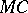which contains the images of spaces in a classunder continuous mappings in a class? 3) Letbe the set of continuous mappings whose domains of definition are spaces in a classand whose ranges are spaces in a class, and letbe some other class of mappings. What are the properties of mappings of the class?

These general statements comprise in particular, the following question: What topological properties are preserved by mappings of one kind or another under transition from a space to its image or inverse image? 1) Every-dimensional space (in the sense of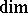) can be mapped essentially (see Essential mapping) onto an-dimensional cube. 2) A pointwise-countable base is preserved under perfect (even under bifactorial) mappings. 3) Every closed mappingin the class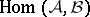, whereis the class of zero-dimensional spaces with a countable base andis the class of-dimensional spaces with a countable base, is at least-fold.

The first specific problems of this kind were solved at the beginning of the 20th century. Such are, for example, the representation of an arbitrary compactum as a continuous image of the Cantor perfect set (Aleksandrov's theorem); the characterization of metric spaces with a countable base as open continuous images of subspaces of the space of irrational numbers (Hausdorff's theorem); the description of locally connected continua as continuous images of an interval. The solution of these problem not only made it possible to answer questions about interrelationships between previously known spaces, but also led to the emergence of interesting new classes of spaces. Such are, for example, dyadic compacta, paracompact-spaces, perfect-dimensional spaces, and pseudo-compact spaces.

The concept of a real-valued continuous function, that is, a continuous mapping of a topological space into, which lies at the basis of the theory of functions, plays an important role also in general topology. Here one must mention first of all the Urysohn lemma, the Brouwer–Urysohn theorem on the extension of continuous functions from closed subsets of normal spaces, A.N. Tikhonov's definition of completely-regular spaces (cf. Completely-regular space), and the Stone–Weierstrass theorem. These and other investigations led to the creation of a theory of rings of continuous functions, the methods of which turned out to be quite fruitful in general topology.

A substantial part of dimension theory is the study of the behaviour of dimensional characterizations of spaces under transition to an image or inverse image by mappings of one class or another. Here an important role is played by-shifts,-mappings,-mappings, essential mappings, finite-to-multiple mappings, countable-to-multiple mappings, zero-dimensional mappings,-dimensional mappings, etc. Here the method of continuous mappings leads to a mutual enrichment of and interrelations between domains of general topology of totally different origin, such as dimension theory, which has an intuitive geometric meaning, and the theory of cardinal invariants, which is abstract in character.

One of the characteristics of dimension is the possibility of extending a continuous mapping from a closed subset to an-dimensional sphere. This is one of the versions of the theorem on the extension of mappings, which, like the fixed-point theorem closely connected with it, is of prime importance in branches of modern mathematics such as topology, algebra, function theory, functional analysis, and differential equations.

One of the best studied classes of continuous mappings is that of the perfect irreducible mappings (cf. Perfect irreducible mapping). The theorem on the absolute of a regular space stimulated entire series of investigations in this domain. In particular, the concept of an absolute has been extended to the class of all Hausdorff spaces. Closely connected with the concept of a continuous mapping turned out to be that of a-proximity, which made it possible to given an intrinsic description of all perfect continuous inverse images of an arbitrary compactum. The extension of the theory of irreducible continuous mappings to the class of all Hausdorff spaces showed that continuous mappings are insufficient for the study of non-regular spaces and that is more natural to consider-continuous mappings in this case.

The selection of uniformly-continuous functions from the class of all numerical functions of one or several variables became one of the starting points of research leading to the notion of a uniform topology.

Continuous mappings of one type or another lie at the basis of the theory of retracts, splines and homology theory. A major role in modern mathematics is played by various aspects of the theory of multi-valued mappings (cf. Multi-valued mapping). Questions related to continuous mappings of Euclidean spaces are interesting by the wealth of ideas they contain.

How to Cite This Entry:
Continuous mapping. V.V. Fedorchuk (originator), Encyclopedia of Mathematics. URL: http://encyclopediaofmath.org/index.php?title=Continuous_mapping&oldid=16175
This text originally appeared in Encyclopedia of Mathematics - ISBN 1402006098